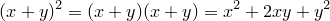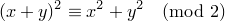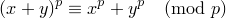## Friday, January 20, 2012

### The Dead Puppy Theorem

So for the past few months I've been telling my kids that, every time they write (x + y)2 = x2 + y2, they kill a puppy.  In fact, I will hereafter refer to that equation as the Dead Puppy Theorem, or DPT.  Since its discovery in early September, my students' usage of the DPT has accounted for more canine deaths than heartworms.

I mean, I get it.  It totally looks like the Dead Puppy Theorem should be true, especially to high school students who have had the distributive property of multiplication over addition hammered into their brains so hard and often that they get points off their social studies papers for trying to multiply  parenthetical citations.  We can hardly blame kids for trying to extend the same type of reasoning to create a perfectly sensible, albeit nonexistent, distributive property of exponentiation over addition.  Well, almost nonexistent.

It's certainly true that the Dead Puppy Theorem is almost always false, which, in the mathematical world, means just plain false, i.e., "not true in every possible case."  (If you want to try it out for yourself, just about any random choice of x and y will provide a counterexample.)  The actual relationship, directly from the definition of "squaring" and the (real) distributive property, looks like this:So it's not the same as the DPT, but it's not so terribly far off from x2 + y2, either: just that pesky little 2xy term.  If we could somehow make it disappear, then we'd indeed be left with the DPT as a result.

One of my students pointed out that, if either x or y is zero, then 2xy = 0, and it's certainly true that, for example, (x + 0)2 = x2 + 02 (ditto for the case where x = 0 instead), but that's not a particularly interesting solution.  What is great about this line of thinking, though, is that it gets us an infinite set of values for which the Dead Puppy Theorem holds.  Of course that's not enough to make it a true statement in general, since there are also an infinite number of counterexamples, but we've produced a special case where the DPT is true, if only trivially.  For a more interesting case, in fact a case where the DPT is true for any natural numbers x and y, we turn to modular arithmetic.

If we look at the DPT modulo 2, we get a different story.  It's still true that (x + y)2 = x2 + 2xy + y2, but since 2xy is congruent to 0 mod 2 (in other words, 2 divides 2xy evenly) we get the following result, the Modified Dead Puppy Theorem:Now, if two numbers are congruent modulo 2, all that really means is that they have the same parity (odd or even), so here is the Modified DPT in plain(ish) English:
The square of the sum of two natural numbers and the sum of their squares are either both even or both odd.
The beauty of this statement, I mean aside from the fact that it looks pleasingly intuitive, is that it has the benefit of actually being true (try out some examples!).

Not satisfied?  We can take this statement even further.  The Modified DPT is really just a special case of a more general theorem, owing to the fact that 2 is a prime number.  In fact, for any choice of prime modulus, p, we have the following Generalized Dead Puppy Theorem:Again, this theorem really is true, though it's a little tougher to couch it in everyday language.  Still, it's beautiful, no?  If you want just a bit of a challenge, it's not that difficult to prove the Generalized DPT using the binomial theorem and some reasoning about factorials, and it's actually sometimes used as a lemma in proving Fermat's Little Theorem.

Here's the point.  It's easy to dismiss student mistakes, or to pay lip service to their pedagogical importance, &c., but asking whether those mistakes are ever right can lead to very cool mathematical conversations, and even to some results that are always right.

Puppies everywhere sleep a little more soundly tonight.

-